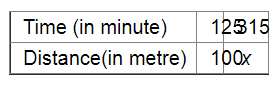# Anupama takes 125 minutes in walking a distance of 100 metre.`
Question:

Anupama takes 125 minutes in walking a distance of 100 metre. What distance would she cover in 315 minutes?

Solution:

Let the distance travelled in 315 minutes be x km.If the distance travelled is more, the time needed to cover it will also be more.

Therefore, it is a direct variation.

We get:

$125: 315=100: x$

$\Rightarrow \frac{125}{315}=\frac{100}{x}$

Applying cross muliplication, we get:

$x=\frac{100 \times 315}{125}$

$=252$

Thus, Anupama would cover 252 metre in 315 minutes.